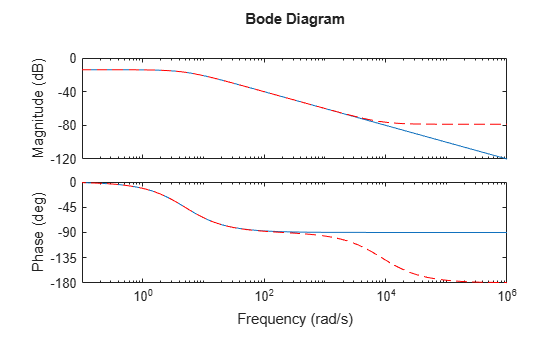# balreal

Gramian-based input/output balancing of state-space realizations

## Syntax

```[sysb,g] = balreal(sys) [sysb,g,T,Ti] = balreal(sys) [___] = balreal(sys,opts) ```

## Description

```[sysb,g] = balreal(sys) ``` computes a balanced realization `sysb` for the stable portion of the LTI model `sys`. `balreal` handles both continuous and discrete systems. If `sys` is not a state-space model, it is first and automatically converted to state space using `ss`.

For stable systems, `sysb` is an equivalent realization for which the controllability and observability Gramians are equal and diagonal, their diagonal entries forming the vector `g` of Hankel singular values. Small entries in `g` indicate states that can be removed to simplify the model (use `modred` to reduce the model order).

If `sys` has unstable poles, its stable part is isolated, balanced, and added back to its unstable part to form `sysb`. The entries of `g` corresponding to unstable modes are set to `Inf`.

```[sysb,g,T,Ti] = balreal(sys) ``` also returns the vector `g` containing the diagonal of the balanced Gramian, the state similarity transformation xb = Tx used to convert `sys` to `sysb`, and the inverse transformation Ti = T-1.

If the system is normalized properly, the diagonal `g` of the joint Gramian can be used to reduce the model order. Because `g` reflects the combined controllability and observability of individual states of the balanced model, you can delete those states with a small `g(i)` while retaining the most important input-output characteristics of the original system. Use `modred` to perform the state elimination.

`[___] = balreal(sys,opts)` computes the balanced realization using options that you specify using `hsvdOptions`. Options include offset and tolerance options for computing the stable-unstable decompositions. The options also allow you to limit the Gramian computation to particular time and frequency intervals. See `hsvdOptions` for details.

## Examples

collapse all

Consider the following zero-pole-gain model, with near-canceling pole-zero pairs:

`sys = zpk([-10 -20.01],[-5 -9.9 -20.1],1)`
```sys = (s+10) (s+20.01) ---------------------- (s+5) (s+9.9) (s+20.1) Continuous-time zero/pole/gain model. ```

A state-space realization with balanced gramians is obtained by

`[sysb,g] = balreal(sys);`

The diagonal entries of the joint gramian are

`g'`
```ans = 1×3 0.1006 0.0001 0.0000 ```

This indicates that the last two states of `sysb` are weakly coupled to the input and output. You can then delete these states by

`sysr = modred(sysb,[2 3],'del');`

This yields the following first-order approximation of the original system.

`zpk(sysr)`
```ans = 1.0001 -------- (s+4.97) Continuous-time zero/pole/gain model. ```

Compare the Bode responses of the original and reduced-order models.

`bodeplot(sys,sysr,'r--')`The plots shows that removing the second and third states does not have much effect on system dynamics.

Create an unstable system.

`sys = tf(1,[1 0 -1])`
```sys = 1 ------- s^2 - 1 Continuous-time transfer function. ```

Apply `balreal` to create a balanced-gramian realization.

`[sysbal,g] = balreal(sys)`
```sysbal = A = x1 x2 x1 1 0 x2 0 -1 B = u1 x1 -0.7071 x2 -0.7071 C = x1 x2 y1 -0.7071 0.7071 D = u1 y1 0 Continuous-time state-space model. ```
```g = 2×1 Inf 0.2500 ```

The unstable pole shows up as `Inf` in the vector `g`.

## Algorithms

Consider the model

`$\begin{array}{l}\stackrel{˙}{x}=Ax+Bu\\ y=Cx+Du\end{array}$`

with controllability and observability Gramians Wc and Wo. The state coordinate transformation $\overline{x}=Tx$ produces the equivalent model

`$\begin{array}{l}\stackrel{˙}{\overline{x}}=TA{T}^{-1}\overline{x}+TBu\\ y=C{T}^{-1}\overline{x}+Du\end{array}$`

and transforms the Gramians to

`$\begin{array}{cc}{\overline{W}}_{c}=T{W}_{c}{T}^{T},& {\overline{W}}_{o}={T}^{-T}{W}_{o}\end{array}{T}^{-1}$`

The function `balreal` computes a particular similarity transformation T such that

`${\overline{W}}_{c}={\overline{W}}_{o}=diag\left(g\right)$`

See ,  for details on the algorithm.

If you use the `TimeIntervals` or `FreqIntervals` options of `hsvdOptions`, then `balreal` bases the balanced realization on time-limited or frequency-limited controllability and observability Gramians. For information about calculating time-limited and frequency-limited Gramians, see `gram` and .

## References

 Laub, A.J., M.T. Heath, C.C. Paige, and R.C. Ward, "Computation of System Balancing Transformations and Other Applications of Simultaneous Diagonalization Algorithms," IEEE® Trans. Automatic Control, AC-32 (1987), pp. 115-122.

 Moore, B., "Principal Component Analysis in Linear Systems: Controllability, Observability, and Model Reduction," IEEE Transactions on Automatic Control, AC-26 (1981), pp. 17-31.

 Laub, A.J., "Computation of Balancing Transformations," Proc. ACC, San Francisco, Vol.1, paper FA8-E, 1980.

 Gawronski, W. and J.N. Juang. “Model Reduction in Limited Time and Frequency Intervals.” International Journal of Systems Science. Vol. 21, Number 2, 1990, pp. 349–376.